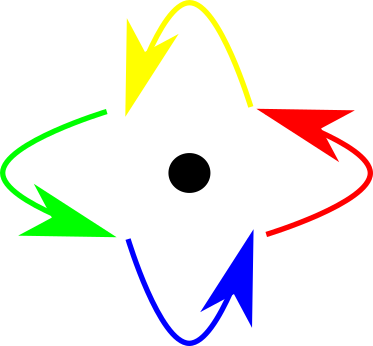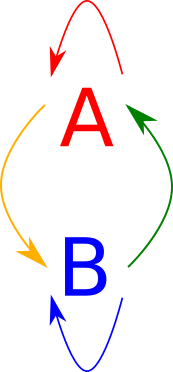# Monoids, Bioids, and Beyond

## Two View of Monoids.

### Monoids

Monoids are defined in Haskell as follows:

class Monoid a where
m_id :: a
(++) :: a -> a -> a


Monoids define some operation with identity (here called m_id). We can define the required laws, identity, and associativity, as follows:

monoidLaw1, monoidLaw2 :: (Monoid a, Eq a) => a -> Bool
monoidLaw1 x = x ++ m_id == x
monoidLaw2 x = m_id ++ x == x

monoidLaw3 :: (Monoid a, Eq a) => a -> a -> a -> Bool
monoidLaw3 x y z = (x ++ y) ++ z == x ++ (y ++ z)


### Morphisms

Now, I’ll introduce something else:

class (Morphism f) where
id :: f x x
(.) :: f b c -> f a b -> f a c


Morphisms also have laws:

morphismLaw1, morphismLaw2 :: (Morphism f, Eq (f a b)) => f a b -> Bool
morphismLaw1 f = f . id == f
morphismLaw2 f = id . f == f

morphismLaw3 :: (Morphism f, Eq (f a d)) => f c d -> f b c -> f a b -> Bool
morphismLaw3 f g h = (f . g) . h == f . (g . h)


This definition defines a morphism, or a generalization of a function, in the category of Haskell types.

We can make things instances of morphisms as such:

instance (Morphism (->)) where
id x = x
(f . g) x = f (g x)


### Morphisms and Monoids

Note that while a Monoid is a concrete type, a Morphism is a higher-order-type that takes two types as inputs. However, apart from this, we have fairly similar set of given functions with a similar set of laws.

To make the comparison explicit, we can look at endomorphisms, that is morphisms from a set to itself.

data Endo morph set = Endo (morph set set)

instance (Morphism morph) => (Monoid (Endo morph set)) where
m_id = Endo id
Endo f ++ Endo g = Endo (f . g)


So, we can see that monoids can be viewed as morphisms from a set to itself. Here is a diagram of that. The elements of the monoid are the arrows.In any case, we now have a new way of expressing a monoid: as a set of morphisms from a set to itself.

## Bioids

We can define a bioid to be the arrows between two objects. These fall into four different sets, those from $$A \to A$$, those from $$A \to B$$, those from $$B \to A$$, and those from $$B \to B$$. This can be represented diagrammatically as follows:If we want to enforce the laws of the category, we know that we need to enforce a monad structure on both $$A$$ and $$B$$. We also know that we must have four additional composition values. In Haskell syntax, we have:

class (Monoid aa, Monoid bb) => Bioid aa bb ab ba
| aa -> ab, aa -> ba, bb -> ab, bb -> ba, ab -> aa, ab -> bb, ba -> aa, ba -> bb, aa -> bb, bb -> aa, ab -> ba, ba -> ab where
id_a :: aa
id_b :: bb
(%%) :: ab -> ba -> aa
(^^) :: ba -> ab -> bb

(#>) :: ab -> aa -> ab
(<#) :: aa -> ba -> ba
($>) :: bb -> ab -> ab (<$) :: ba -> bb -> ba


OK, I don’t want to write down the laws for that mess. I now understand why mathematicians stopped at monoids but not bioids. To be completely honest, I was hoping to get semirings out of this mess, but I don’t think it’s actually possible.

## And Beyond

In any case, we can still notice something interesting about a Bioid. It has a total of eight composition rules, if you include the two monoid rules. How can we calculate this number in general?

First, let’s define an n-oid as the morphisms on a category with $$n$$ objects. We know that there are $$n^2$$ types of morphisms ($$n$$ sources and $$n$$ destinations). We also know that each composition rule contains three types in any order:

$$\circ :: m b c \to m a b \to m b c$$

So there are therefore $$n^3$$ ++ equivalents on an n-oid. Yeah, in general that’s going to get messy very fast.